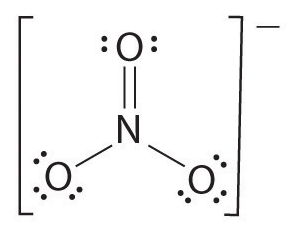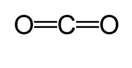# How to Calculate Bond Order and Bond Length

Determining certain parameters such as size, length, and angle in atomic scale is not easy. Because of the important of these parameters, scientists have developed methods to deduce or calculate the parameters in atomic level. Bond order and bond length are two such important parameters that indicate the type and strength of bonds between a pair of atoms.

1. What are Bond Order and Bond Length

2. How to Calculate Bond Order and Bond Length
– How to Calculate Bond Order
– How to Calculate Bond Length

## What are Bond Order and Bond Length

Bond length and bond order are two parameters that are associated with covalent bonds. Bond order is the number of chemical bonds between two atoms and bond length is the distance between two nuclei of atoms that are covalently bonded together. This article explains how to calculate bond order and bond length in an atomic level.

## How to Calculate Bond Order

Bond order is the number of chemical bonds between two atoms. It indicates the stability of a bond. In covalent bonds, the bond order is the number of shared electrons. For example, bond order of a pair of atoms bonded by a single bond is one, whereas bond order of a pair of atoms bonded by a double bond is two. Zero bond order indicates that there is no bond between atoms. The stability of a molecule increases with increasing bond order. The molecules with resonance bonding do not need to be an integer. In covalent compounds with two atoms, the bond order between a pair of atoms is determined by first drawing the Lewis structure, and then determining the type of bonds between the atoms – zero bond, single, double or triple bond. For example, the bond order of hydrogen gas is determined as follows.

1. Draw the Lewis structure

H: H

1. Determine the number of bonds/pair of valence electrons

One pair of electrons, hence the bond order is 1.

If there are more than two atoms, the bond order is determined as follows. See the example: nitrate ion.

1. Draw the Lewis structure2. Count the total number of bonds (according to nitrate ion, its 4)
1. The number of bond groups between individual atoms (according to ammonia, its 3)
1. Therefore, the bond order    = Total number of bonds/ number of bond groups

= 4/3

= 1.33

Therefore, the bond order of nitrate ion is 1.33

## How to Calculate Bond Length

Bond length is the distance between two nuclei of atoms that are covalently bonded together. Bond length is usually in the range of 0.1 to 0.2 nm. When two similar atoms are bonded together, half of the bond length is referred to as covalent radius. Bond length depends on the number of bonded electrons of two atoms or the bond order. Higher the bond order, shorter the bond length will be due to strong pulling forces of positively charged nuclei. The unit of bond length is picometer. In single, double and triple bonds, the bond length increases in the order of

triple bond<double bond<single bond

Electronegativity can be used to calculate bond length between two atoms having different electronegativities. The following empirical formula was proposed by Shoemaker and Stevenson to calculate bond length.

dA-B = rA + rB – 0.09 (xA – xB)

dA-B is bond distance between two atoms A and B, rA and rB are covalent radii of A and B, and

(xA – xB) is electronegativity difference between A and B.

Another method is used to calculate the approximate bond length. In this method, first Lewis structure is drawn to determine the covalent bond type. Then using a chart* made on the studies done by Cordero et al., and Pyykkö and Atsumi, the corresponding radii of the bonds made by each atom are determined. Then the bond length is determined by taking the sum of the two radii. For example, when determining the bond length of carbon dioxide, first Lewis structure is drawn.According to the chart, the covalent radius of carbon double bond is 67 picometers and that of oxygen double bond is 57 picometers. Therefore, the bond length of carbon dioxide is approximately 124 picometers (57 pm+ 67 pm).

*Covalent chart is found here

Reference:

Cordero, Beatriz, et al. “Covalent radii revisited.” Dalton Transactions 21 (2008): 2832-2838.

Libretexts. “Bond Order and Lengths.” Chemistry LibreTexts. Libretexts, 05 Dec. 2016. Web. 10 Jan. 2017.

Lister, Ted, and Janet Renshaw. Understanding Chemistry for Advanced Level. N.p.: Nelson Thornes, 2000. Print.

Pratiyogita Darpan. “Properties of Elements in Relation to their Electronic Structure .” Competition Science Vision Aug. 1998: n. pag. Print.

Pyykkö, Pekka, and Michiko Atsumi. “Molecular Double‐Bond Covalent Radii for Elements Li–E112.” Chemistry–A European Journal 15.46 (2009): 12770-12779.